Next: Functions Up: Elements of the Fib Previous: Comment element   Contents   Index

## Area element

The area element defines a variable. It sets the defined variable to integers of a specified range of integers. The area element contains, in addition to the defined variables and the subobject to which it applies, a list of areas, which the variable will go through. The variable is valid everywhere in the subobject.

Syntax: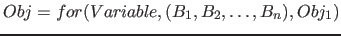Description of the elements:

•: The variable, which the area element defines.
•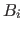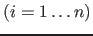are the areas. For the value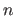is, so there is at least one subarea in the area element.
•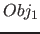: The subobject, for which theis defined and which will be evalued for every variable assignment of the area.

One (sub-)areais a vector of degree 2, whose two integer components specify an integer field, to which the variable will be set. An areaconsists of the two (integers) elements of the vectorand all integers between them. If one element of the vector is a variable, that contains a non-integer value, it is rounded to an integer. For the rounding, the decimal digit befor the point remains the same, if the first decimal digit after the point is between 0 to 4, otherwise (from 5 to 9) the number of the decimal befor the point is increased by one for positive numbers and decreases by one for negative numbers.

The area element includes as its area the union of all its subareas. It will therefore go through a range of all integers, which are contained in its subareas.

Examples:

•; In this example, the variablefor the object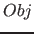is set to the successive values: 1; 2; 3; 10; 11; 12; 13; 14
•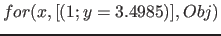; In this example, the variablefor the objectis set to the successive values: 1; 2; 3
•; In this example, the variablefor the objectis set to the successive values: 1; 2; 3; 4

Note: With this definition of the area element continuous functions can not be realized, because the area element just allows integers and dosn't allow continuous transition of values. Functions can only be realized continuous up to a certain point (e. g. in the range of integers).

Example: The functionfor the area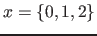(chosen simple just for clarification)

If it is tried to realize it in the form: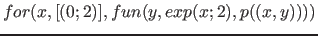gaps will result (in the transition from (1, 1) to (2, 4) a point (x, 3) is missing).

However, this can be solved by "compression of the range":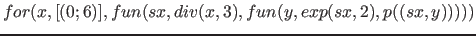Now a point (2;3) exists (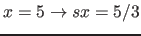rounded up;rounded up)

This has been choosen in favor for an easier implementation and better performance. Multimedia objects (e. g. images) which are encoded in the Fib multimedia description language, will be made of individual dots (pixels) in the representation.

However, it is possible to achieve a scalability of a Fib multimedia object in other ways.Next: Functions Up: Elements of the Fib Previous: Comment element   Contents   Index
Betti Österholz 2013-02-13